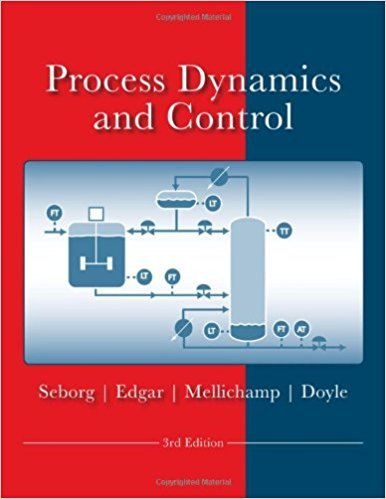×
Get Full Access to Process Dynamics And Control - 3 Edition - Chapter 5 - Problem 5.5
Get Full Access to Process Dynamics And Control - 3 Edition - Chapter 5 - Problem 5.5

×

# A thermocouple has the following characteristics when itISBN: 9780470128671 148

## Solution for problem 5.5 Chapter 5

Process Dynamics and Control | 3rd Edition

• Textbook Solutions
• 2901 Step-by-step solutions solved by professors and subject experts
• Get 24/7 help from StudySoup virtual teaching assistantsProcess Dynamics and Control | 3rd Edition

4 5 1 264 Reviews
22
5
Problem 5.5

A thermocouple has the following characteristics when it is immersed in a stirred bath: Mass of thermocouple = 1 g Heat capacity of thermocouple = 0.25 cal!g oc Heat transfer coefficient = 20 cal!cm2h oc (for thermocouple and bath) Surface area of thermocouple = 3 cm2 (a) Derive a transfer function model for the thermocouple relating the change in its indicated output T to the change in the temperature of its surroundings Ts assuming uniform temperature (no gradients in the thermocouple bead), no conduction in the leads, constant physical properties, and conversion of the millivolt-level output directly to a oc reading by a very fast meter. (b) If the thermocouple is initially out of the bath and at room temperature (23 oq, what is the maximum temperature that it will register if it is suddenly plunged into the bath (80 oq and held there for 20 s?

Step-by-Step Solution:

Problem 5.5A thermocouple has the following characteristics when it is immersed in a stirred bath: Mass of thermocouple = 1 g Heat capacity of thermocouple = 0.25 cal/g °C Heat transfer coefficient = 20 cal/cm h °C (for thermocouple and bath) Surface area of thermocouple = 3 cm 2(a) Derive a transfer function model for the thermocouple relating the change in its indicatedoutput T to the change in the temperature of its surroundings Ts assuming uniform temperature(no gradients in the thermocouple bead), no conduction in the leads, constant physicalproperties, and conversion of the millivolt-level output directly to a oc reading by a very fastmeter.(b) If the thermocouple is initially out of the bath and at room temperature (23 °C), what is themaximum temperature that it will register if it is suddenly plunged into the bath (80 °C) and heldthere for 20 s Step-by-step solution Step 1 of 7 ^(a)Consider the energy balance for the thermocouple. dT mC dt = hA(T sT)Here,m is mass of thermocouple,C is the heat capacity of thermocouple ,h is the heat transfer coefficient,A is surface area of thermocouple, andt is the time is sec.

Step 2 of 7

Step 3 of 7

##### ISBN: 9780470128671

The full step-by-step solution to problem: 5.5 from chapter: 5 was answered by , our top Engineering and Tech solution expert on 11/15/17, 04:03PM. This full solution covers the following key subjects: thermocouple, temperature, bath, output, CAl. This expansive textbook survival guide covers 24 chapters, and 420 solutions. This textbook survival guide was created for the textbook: Process Dynamics and Control, edition: 3. Process Dynamics and Control was written by and is associated to the ISBN: 9780470128671. The answer to “A thermocouple has the following characteristics when it is immersed in a stirred bath: Mass of thermocouple = 1 g Heat capacity of thermocouple = 0.25 cal!g oc Heat transfer coefficient = 20 cal!cm2h oc (for thermocouple and bath) Surface area of thermocouple = 3 cm2 (a) Derive a transfer function model for the thermocouple relating the change in its indicated output T to the change in the temperature of its surroundings Ts assuming uniform temperature (no gradients in the thermocouple bead), no conduction in the leads, constant physical properties, and conversion of the millivolt-level output directly to a oc reading by a very fast meter. (b) If the thermocouple is initially out of the bath and at room temperature (23 oq, what is the maximum temperature that it will register if it is suddenly plunged into the bath (80 oq and held there for 20 s?” is broken down into a number of easy to follow steps, and 147 words. Since the solution to 5.5 from 5 chapter was answered, more than 1118 students have viewed the full step-by-step answer.

Unlock Textbook Solution